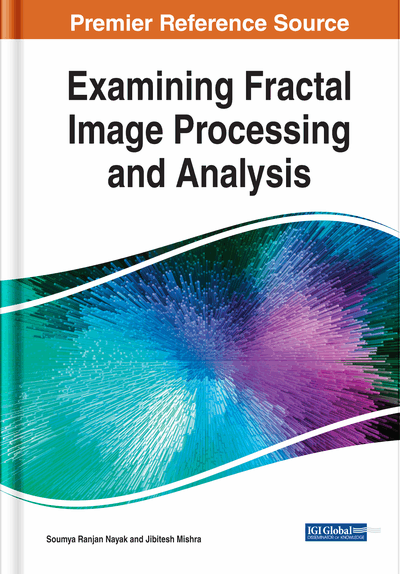# An Edge Detection Approach for Fractal Image Processing

Kalyan Kumar Jena, Sasmita Mishra, Sarojananda Mishra
DOI: 10.4018/978-1-7998-0066-8.ch001
OnDemand:
(Individual Chapters)
Available
\$29.50
No Current Special Offers

## Abstract

Research in the field of fractal image processing (FIP) has increased in the current era. Edge detection of fractal images can be considered as an important domain of research in FIP. Detecting edges in different fractal images accurate manner is a challenging problem in FIP. Several methods have introduced by different researchers to detect the edges of images. However, no method works suitably under all conditions. In this chapter, an edge detection method is proposed to detect the edges of gray scale and color fractal images. This method focuses on the quantitative combination of Canny, LoG, and Sobel (CLS) edge detection operators. The output of the proposed method is produced using matrix laboratory (MATLAB) R2015b and compared with the edge detection operators such as Sobel, Prewitt, Roberts, LoG, Canny, and mathematical morphological operator. The experimental outputs show that the proposed method performs better as compared to other traditional methods.
Chapter Preview
Top

## Introduction

A fractal is a geometric shape (non regular) which has the equal degree of non regularity on every scale. It is considered as a fragmented or rough geometric structure which can be split into parts each of which is recognized as a reduced size replication of the whole. Several concepts and techniques of FIP are provided by Krantz et al. (1989), Uemura et al. (2000), Falconer (2004), Bassingthwaighte et al. (2013), Russ (2013), Nayak et al. (2015), Nayak et al. (2016), Nayak et al. (2018a), Nayak et al. (2018b), Nayak et al. (2018c), Nayak et al. (2018d), Nayak et al. (2018e), Nayak et al. (2018f), Nayak et al. (2018g), Nayak et al. (2019), Bhatnagar et al. (2019), Joardar et al. (2019), Kadam et al. (2019), Yin et al. (2019), Joshi et al. (2019), Li et al. (2019) and Padmavati et al. (2019). Fractals are considered as never ending patterns. These are referred to as infinitely complex patterns which are self similar across several scales. It is required to repeat a simple process again and again in an ongoing feedback loop in order to create the fractals. From mathematics point of view, a fractal is considered as a subset of Euclidean space for which the hausdorff dimension exceeds (strictly) the topological dimension. Fractals have the tendency to appear nearly equal at several levels. These provide identical patterns at increasingly small scales which are considered as unfolding symmetry or expanding symmetry. When the replication is same (exactly) at every scale, then it is referred to as affine self similar. On the basis of mathematical equations, these are normally nowhere differentiable. Generally, these are not limited to geometric patterns and also describe processes in time. Different techniques such as iterated function systems, L-systems, finite subdivision rules, etc. can be used to generate fractals. Fractal generating programs can be used to create fractal images. These can be used for modelling natural structures, image compression, analysis of medical diagnostic images, study of chaotic phenomenon, study of convergence of iterative processes, etc. Fractal geometry can be used to approximate several natural objects to a certain degree including mountain ranges, clouds, vegetables, coastlines, etc. These geometry concepts can be used to simulate as well as understand several objects in nature. Different methods or operators such as Sobel, Prewitt, Roberts, LoG, Canny, mathematical morphological operator, etc. can be used to detect the edges of fractal images. Several approaches, concepts as well as techniques are provided by Gonzalez et al. (2004), Gonzalez et al. (2007), Wang et al. (2016), Xin et al. (2012), Lahani et al. (2018), Othman et al. (2017), Podder et al. (2018), Shanmugavadivu et al. (2014), Wan et al. (2007), Wang (2007), Patel et al. (2011), Coleman et al. (2004), Huertas et al. (1986), Gupta et al. (2013), Chaple et al. (2014), Alshorman et al. (2018), Agrawal et al. (2018), Avots et al. (2018), Halder et al. (2019), Goel et al. (2013), Katiyar et al. (2014), Yu-qian et al. (2006), Giannarou et al. (2005), Scharcanski et al. (1997), Hemalatha et al. (2018) and Kumar et al. (2017) related to edge detection of several images.

The main contribution in this chapter is mentioned as follows:

## Key Terms in this Chapter

Fractal: A geometric shape (non-regular).

Roberts Operator: An edge detection operator that focuses on approximation of image gradient using discrete differentiation operation.

Canny Operator: An edge detection operator that focuses on multistage algorithm for detecting edges in several images.

CLS: Canny, LoG, and Sobel.

Prewitt Operator: An edge detection operator that focuses on the computation of gradient approximation of the image intensity function.

LoG Operator: An edge detection operator that focuses on zero crossings points in several images.

Mathematical Morphology: A technique to analyze and process geometrical structures.

Sobel Operator: An edge detection operator that focuses on two dimensional gradient measurements on several images and highlights the high spatial frequency regions which correspond to edges.

Fractal Image Processing: Application of computer algorithms for performing image processing on several fractal images.

Edge Detection: An image processing technique to find the objects boundaries within images.

## Complete Chapter List

Search this Book:
Reset